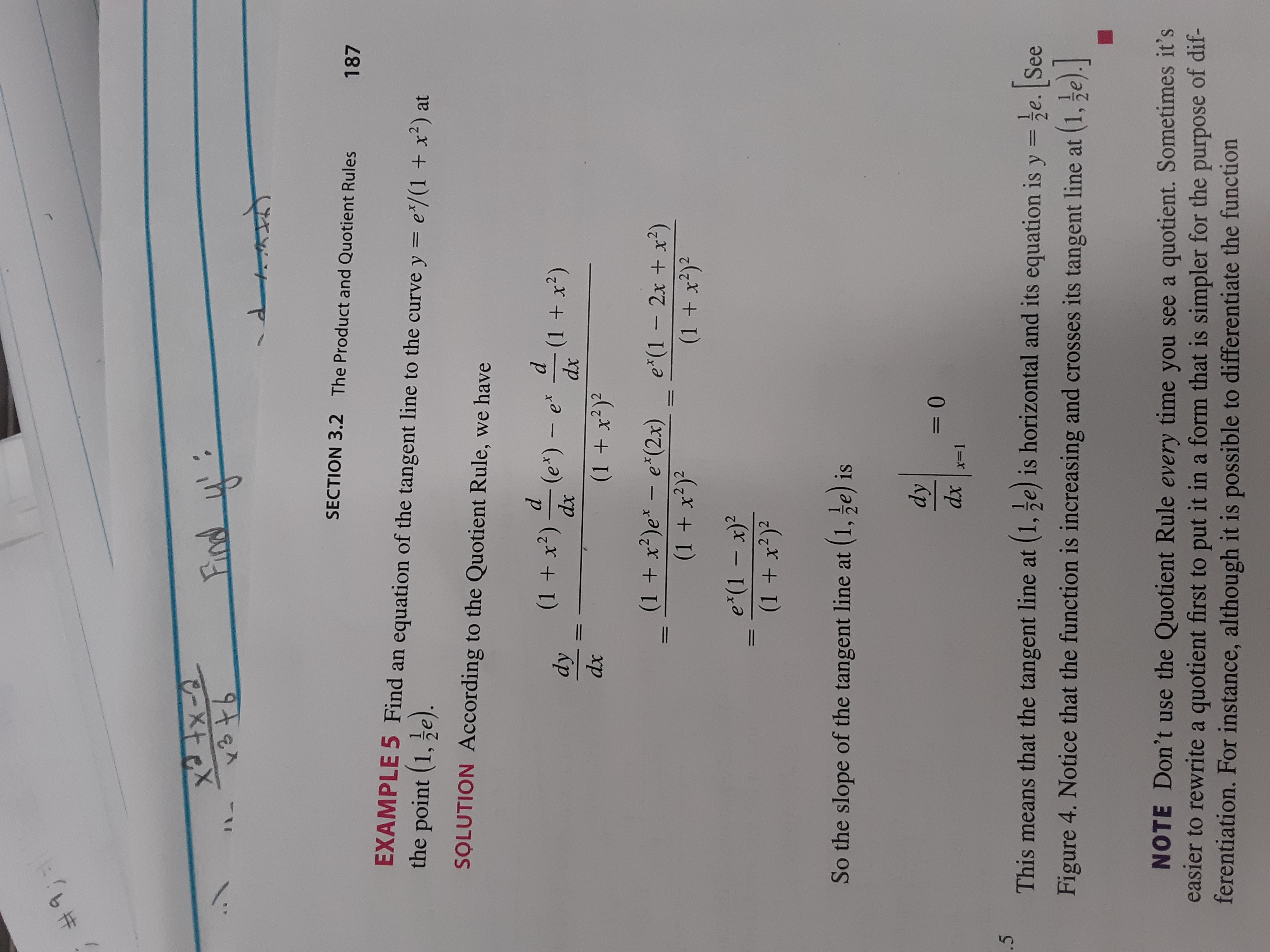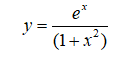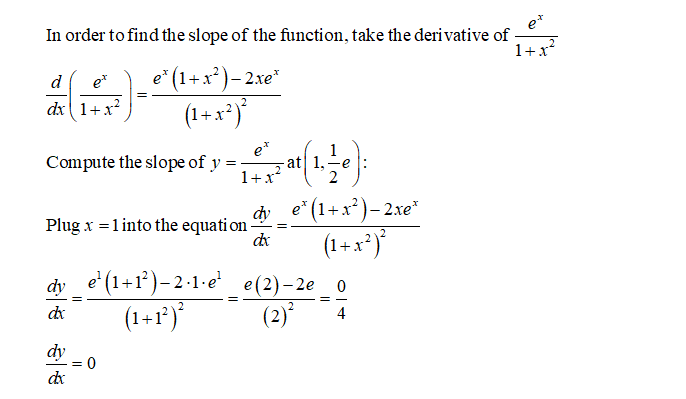# #9;तिलाोSECTION 3.2The Product and Quotient Rules187EXAMPLE 5 Find an equation of the tangent line to the curve y = e*/(1 + x²) atthe point (1, e).SOLUTION According to the Quotient Rule, we have(1 + x')(e*) – e*dx(1 + x²)dxdydx(1 + x?)?(1 + x²)e* - e*(2x)e*(1 – 2x + x²)(1 + x?)²(1 + x²)²e*(1 – x)?(1 + x²)²%3DSo the slope of the tangent line at (1, ze) isdydxx=1.5This means that the tangent line at (1, e) is horizontal and its equation is y =e. See%3DFigure 4. Notice that the function is increasing and crosses its tangent line at (1, 5e).NOTE Don't use the Quotient Rule every time you see a quotient. Sometimes it'seasier to rewrite a quotient first to put it in a form that is simpler for the purpose of dif-ferentiation. For instance, although it is possible to differentiate the function1.

Question
6 views

This is an example from the text. Can you show me how to get the slope? I do not fully understand ((dy)/(dx))|x=1 =0...help_outlineImage Transcriptionclose#9; तिला ो SECTION 3.2 The Product and Quotient Rules 187 EXAMPLE 5 Find an equation of the tangent line to the curve y = e*/(1 + x²) at the point (1, e). SOLUTION According to the Quotient Rule, we have (1 + x') (e*) – e* dx (1 + x²) dx dy dx (1 + x?)? (1 + x²)e* - e*(2x) e*(1 – 2x + x²) (1 + x?)² (1 + x²)² e*(1 – x)? (1 + x²)² %3D So the slope of the tangent line at (1, ze) is dy dx x=1 .5 This means that the tangent line at (1, e) is horizontal and its equation is y =e. See %3D Figure 4. Notice that the function is increasing and crosses its tangent line at (1, 5e). NOTE Don't use the Quotient Rule every time you see a quotient. Sometimes it's easier to rewrite a quotient first to put it in a form that is simpler for the purpose of dif- ferentiation. For instance, although it is possible to differentiate the function 1. fullscreen
check_circle

Step 1 Given:Step 2 Calculation:...

### Want to see the full answer?

See Solution

#### Want to see this answer and more?

Solutions are written by subject experts who are available 24/7. Questions are typically answered within 1 hour.*

See Solution
*Response times may vary by subject and question.
Tagged in

### Calculus